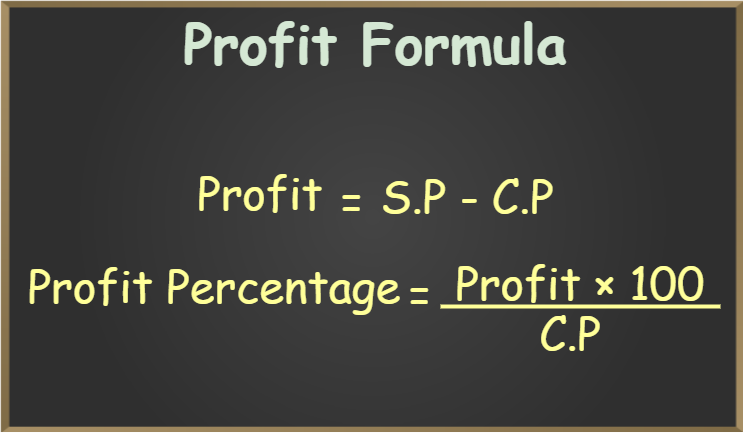Open in App
Not now

# Profit Formula

• Last Updated : 09 Nov, 2022

Profit is defined as the amount obtained by selling a product that is greater than the cost price of the product. It is the amount gained from any type of commercial activity. In summary, if the selling price (SP) of a product is greater than the cost price (CP), it is deemed a gain or profit. It explains the financial gain achieved when the revenue from a commercial activity surpasses the taxes, costs, and so on that are involved in the maintenance of a firm.

## Profit Formula

Profit is better described in terms of cost price and selling price. The cost price of a product or commodity is its real price, whereas the selling price is the amount at which the object is sold. So, if the selling price of the product exceeds the cost price, the firm has made a profit.

As a result, the profit calculation formula is as follows,

Profit = S.P. – C.P.

where,

C.P. is the cost price of the goods
S.P. is the selling price of the goods

## Formulas to Calculate Profit

The various formulas to calculate Profit Formulas are:

• Formula for Profit Percentage is dependent on Profit and C.P.

Profit Percent Formula = (Profit × 100)/ C.P.

• Gross Profit Formula is the profit on the total sales of goods

Gross Profit = Total Revenue – Total Cost of Goods Sold

• Net Profit is the profit that is incurred after excluding all expenses and other costs.

Net Profit = Gross profit – Expenses

## Profit Percentage

Profit percentage (%) is the amount of profit represented as a percentage of the total. Because this profit is dependent on the cost price.

Profit percentage = (Profit / Cost price ) × 100## Solved Examples on Profit Formula

Example 1: A shopkeeper buys pens in bulk for Rs. 20 each. He sells each of them for Rs. 45. What will be the profit and the profit percentage?

Solution:

Given: Selling price of the Pen (S.P) = Rs. 45

Cost price of the Pen (C.P) = Rs. 20

Now, As per the profit formula,

Profit = Selling Price – Cost Price

So, profit = 45 – 20

Profit = Rs. 25

Now, Using the formula for profit percentage,

Profit % = (Profit / Cost Price ) × 100

So, the profit percentage = (25 / 20) × 100

= 1.25 × 100

Profit percentage = 125%.

Example 2: If a shopkeeper sells fruits at Rs.250 per kg, whose cost price is Rs.150/- per kg. What will be the profit gained by the shopkeeper?

Solution:

Given, Cost Price = Rs.150/-

Selling Price = Rs.250/-

From the formula of profit, we know,

Profit = Selling Price – Cost Price

Profit = 250 – 150

Profit = 100

Therefore, the shopkeeper earn profit Rs. 100/- .

Example 3: Find the gain percentage if the profit is Rs 100 and the cost price is Rs 150.

Solution:

As we know,

The profit percentage formula is

Profit percentage % = (Profit / Cost Price ) × 100

Since, Profit  = Rs 100 and Cost Price = Rs 150

Therefore, Profit % = (100/150) × 100

Profit % = 10000/150

= 66.66

Hence, the gain percentage is 66.66%.

Example 4: Sanju sold a digital watch for Rs. 6,000, on which he gains 25%. What is the cost price of the digital watch?

Solution:

For the digital watch Gain = 25%.

Let cost price of watch (C.P.) = Rs.100.

Therefore, selling price (S.P.) of digital watch = (100 + 25) = 125

When selling price (S.P.) is Rs.125, cost price (C.P.) is Rs.100.

Therefore, when selling price (S.P.) is Rs.6000,

Cost price (C.P.) = {100 / (100 + gain )} × 6000

= 100/125 × 6000

= (100 × 6000)/125

= 600000/125

= 4800

Therefore, cost price (C.P.) of the digital watch = Rs. 4800.

Example 5: A cost price for the bag is Rs. 350 and the selling price is Rs. 400. Find the profit percentage he earned.

Solution:

Cost price = Rs 350

Selling price = Rs 400

Profit = SP – CP

= 400 – 350

= 50.

Gain% = (Profit / Cost price ) x 100

= (50/350) × 100

= 14.28 %

Example 6: What will be the selling price if the profit is Rs 100 and the cost price is Rs 350?

Solution:

Let selling price = x

Given cost price = Rs 350

And profit = Rs 100

Profit = Selling Price (S.P)  – Cost Price (C.P)

100 = x  – 350

or x = 100 + 350

x = 450

So the selling price is Rs 450.

## FAQs on Profit Formula

Question 1: What is the formula to calculate the profit?

Formula for calculating the profit is given by, Profit = Selling Price – Cost Price.

Question 2: What is the formula to calculate the loss?

Formula for calculating the loss is given by, Loss = Cost Price – Selling Price.

Question 3: What is the Profit Percentage Formula?

Formula for calculating the profit percentage is given by Profit Percentage = (Profit / C.P.) × 100

Question 4: What is Profit Formula?

If a product is sold at a higher price than its cost price a profit is earned. The profit earned is calculated by the profit formula. The profit formula is given as, Profit = S.P. – C. P.

Question 5: What is the Profit Percentage Formula using the Selling Price?

Steps for finding profit percentage if S.P. and Profit are given:

Step 1: Calculate C.P. by formula C.P. = S.P. – Profit

Step 2: Using C.P. and Profit calculate the profit percentage by the formula

Profit Percentage = (Profit / C.P.) × 100

Thus, the profit percentage is calculated.

### Related Resources

My Personal Notes arrow_drop_up
Related Articles